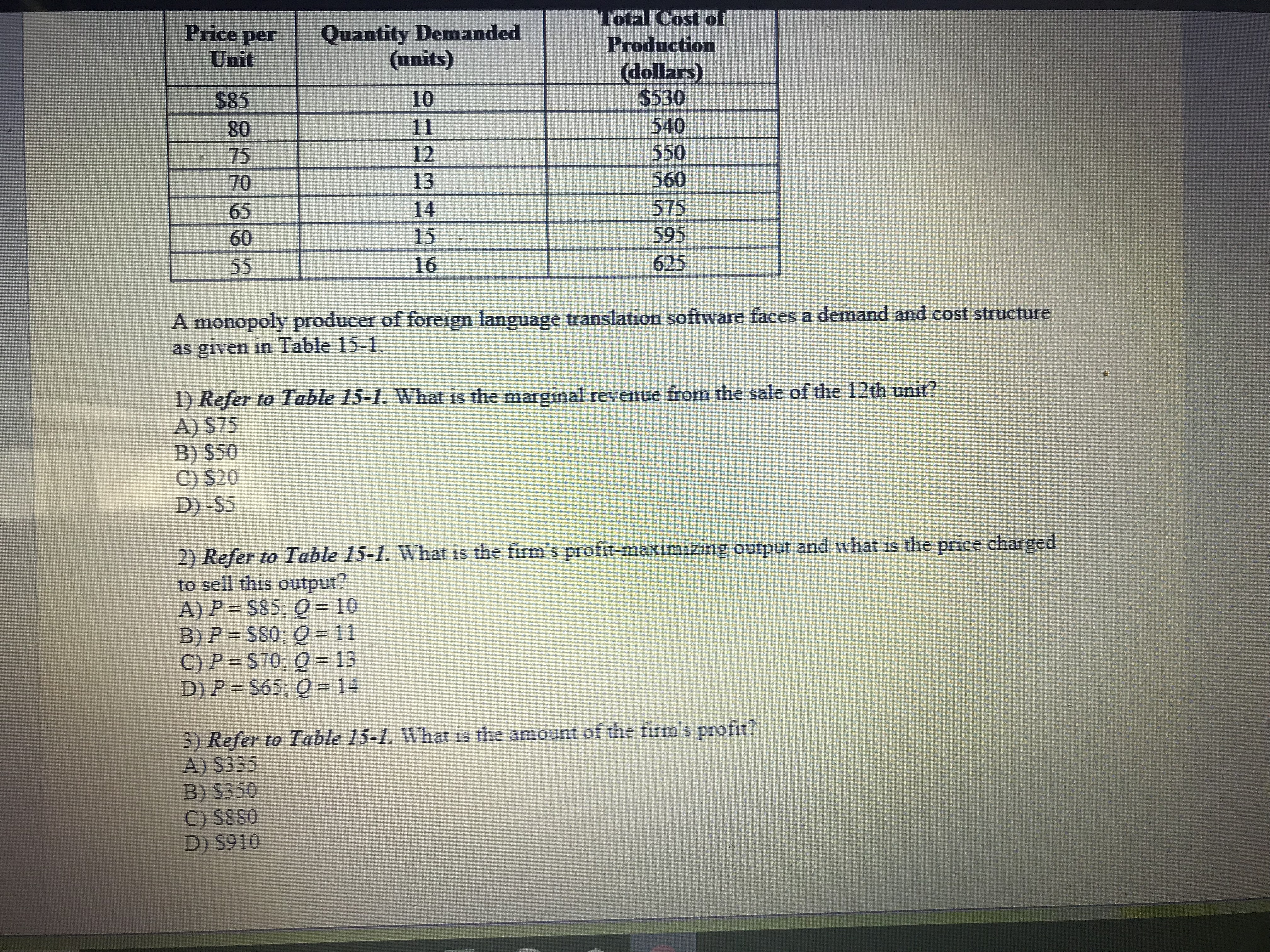ToalCost ofPrice per Quantity DemandedProduction(dollars)\$530540550560575595625Unit(units)\$858075706560101213141516A monopoly producer of foreign language translation software faces a demand and cost structureas given in Table 15-1.1) Refer to Table 15-1. What is the marginal revenue from the sale of the 12th unit?A) S75B) S50C) S20D) -S52) Refer to Table 15-1. What is the firm's profit-maximizing output and what is the price chargedto sell this output?A) P S85: 0 10B) P-380; Q = 11C) P S70: Q 13D)P- S65: 0-143) Refer to Table 15-1. What is the amount of the firm's profit?A) S335B) S350C) S880D) \$910

Question

How do you do number 1help_outlineImage TranscriptioncloseToalCost of Price per Quantity Demanded Production (dollars) \$530 540 550 560 575 595 625 Unit (units) \$85 80 75 70 65 60 10 12 13 14 15 16 A monopoly producer of foreign language translation software faces a demand and cost structure as given in Table 15-1. 1) Refer to Table 15-1. What is the marginal revenue from the sale of the 12th unit? A) S75 B) S50 C) S20 D) -S5 2) Refer to Table 15-1. What is the firm's profit-maximizing output and what is the price charged to sell this output? A) P S85: 0 10 B) P-380; Q = 11 C) P S70: Q 13 D)P- S65: 0-14 3) Refer to Table 15-1. What is the amount of the firm's profit? A) S335 B) S350 C) S880 D) \$910 fullscreen
Step 1

It is given that,

The above table depicts the demand and cost structures of foreign language translator software of a monopoly producer.

To find: The MR of 12th unit, the firm’s profit maximization price and output, and the amount of profit

We know that,

Marginal Revenue is the additional revenue that a firm can generate by selling an additional unit of output in the market; therefore, the MR of 12th unit can be derived by subtracting the 11th unit Total Revenue (TR) from 12th unit TR i.e.  TR (12th unit) – TR (11th unit). Thus, referring to the modified table 15-1, we can write:

MR (12th unit) = \$900 - \$880

= \$20

Therefore, for the above question (1) the correct option is (C).

Step 2

Now, we have to determine the profit maximizing price and output

We know that,

At the profit maximizing level, the amount of profit is maximum; therefore, referring to the modified table 15-1 we can conclude that the profit is maximum (\$350) at two prices i.e. \$70 and \$75 where the profit-maximizing quantities are 13 and 12 units respectively. Ho...

Want to see the full answer?

See Solution

Want to see this answer and more?

Our solutions are written by experts, many with advanced degrees, and available 24/7

See Solution
Tagged in